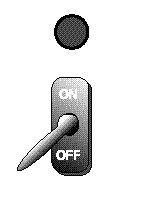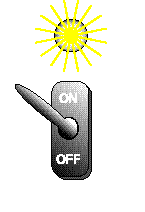# 3.5. Bit Patterns¶

A bit can only represent two possible values - 0 or 1. With one bit there are two possible patterns. How many patterns can be formed with two bits? Here is a complete list:

0 0
0 1
1 0
1 1Try clicking these switches. With two on/off switches, you should be able to make 4 different combinations of on and off.How many patterns can be formed with three bits? Let’s list them:

0 0 0
0 0 1
0 1 0
0 1 1
1 0 0
1 0 1
1 1 0
1 1 1

The list of patterns for three bits has 8 lines (patterns). Notice that the first four patterns are a “0” followed by the possible patterns for 2 bits. The second four patterns are a “1” followed by the patterns for 2 bits.

To form the list of patterns for 4 bits, we can use the same trick. Make two copies of the list for 3 bits. This gives you 16 lines. Put a “0” at the start of the first 8 lines and a “1” at the start of the second 8 lines.

This trick can be repeated as many times as you like. Adding one more bit doubles the number of patterns. The table shows the number of patterns for 1, 2, 3 and 4 bits.

Number of Bits Number of Patterns Number of Patterns as a power of two:

Bits

Patterns

Power of two

1

2

$$2^1$$

2

4

$$2^2$$

3

8

$$2^3$$

4

16

$$2^4$$

How many patterns with 5 bits? Make two copies of the 4-bit patterns (16 patterns per copy). Make the patterns unique by prefixing “0” to the first 16 patterns and “1” to the second 16. You now have $$16×2 = 32 = 2^5$$ unique patterns. This demonstrates the following:

Number of possible patterns of N bits = $$2^N$$

Memorize this fact. Better yet, make lists of patterns (as above) and play around until you understand. Do this now. This is an essential fact. If you allow yourself to get muddled on it, you will waste much time in this and future courses.